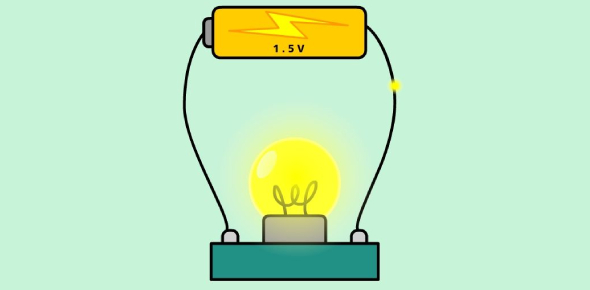# Electricity Exam: Physics Trivia Quiz!

25 Questions | Total Attempts: 98Settings.

• 1.
An electrical appliance has a resistance of 25 Ω. When this electrical appliance is connected to a 230 V supply line, the current passing through it will be:
• A.

3.2A

• B.

4.2A

• C.

9.2A

• D.

8.2A

• 2.
In the given figure, the resistors:
• A.

6 Ω, 3 Ω and 9 Ω are in series

• B.

9 Ω and 6 Ω are in parallel and the combination is in series with 3 ohm

• C.

3 Ω, 6 Ω and 9 Ω are in parallel

• D.

3 Ω and 6 Ω are in parallel and the combination is in series with 9 Ω

• 3.
Electric power is inversely proportional to:
• A.

Resistance

• B.

Voltage

• C.

Current

• D.

Temperature

• 4.
Three resistors of 1 Ω, 2 ohms,s, and 3 Ω are connected in parallel. The combined resistance of the three.
• A.

Equals 3 Ω

• B.

Less than 2Ω

• C.

Equals 2 Ω

• D.

Mor than 3 Ω

• 5.
Point to be kept in mind for verification of Ohm’s Law is:
• A.

Ammeter and voltmeter should be connected in series

• B.

Ammeter should be connected in series and voltmeter in parallel

• C.

Ammeter should be connected in parallel and voltmeter in series

• D.

Ammeter and voltmeter should be connected in parallel

• 6.
There is the wire of length I and cross-section A. Which of the given have the least resistance.
• A.

Length doubled, Area halved

• B.

Length tripled, Area doubled

• C.

Length halved, Area doubled

• D.

The original wire

• 7.
A car headlight bulb working on a 12 V car battery draws a current of 0.5 A. The resistance of the light bulb is:
• A.

0.5 Ω

• B.

B. 6 Ω

• C.

12 Ω

• D.

24Ω

• 8.
The resistance of a conductor depends directly on its ……… and ...... , inversely on its ………
• 9.
According to ohm's Law, the ............ across the ends of a resistor is directly proportional to the ……… flowing through it, provided its ........... remains constant.
• 10.
If the amount of electric charge passing through a conductor in 10 minutes is 300 C, the current flowing is:
• A.

2A

• B.

0.5A

• C.

300A

• D.

30A

• 11.
An electric fuse works on the:
• A.

Chemical effect of current

• B.

Magnetic effect of current

• C.

Lightning effect of current

• D.

Heating effect of current

• 12.
From the graph:
• A.

Electric power

• B.

Voltage

• C.

Resistivity

• D.

Resistance

• 13.
Calculate Resistance.
• A.

150 Ω

• B.

1.5 Ω

• C.

11.5 Ω

• D.

15 Ω

• 14.
If in the given arrangement, the three resistors are to be replaced by a single resistor. What will be the value of this resistor?
• A.

6 Ω

• B.

18 Ω

• C.

12 Ω

• D.

• 15.
In An Electrical Circuit Three Incandescent Bulbs A, B And C Of Rating 40 W, 60 W And 100 W Respectively Are Connected In Parallel To An Electric Source. Which Of The Following Is Likely To Happen Regarding Their Brightness?
• A.

All the three bulbs glow with same brightness

• B.

Bulb A glows with more brightness than bulb B and C

• C.

Bulb C glows with more brightness than A and B

• D.

Bulb B glows with more brightness than A and B

• 16.
When a current 'I' flows through a resistance 'R' for time 't' the electrical energy spent is given by:
• A.

I 2 Rt

• B.

IRt

• C.

2 It

• D.

2 RI

• 17.
Which one connected properly?
• A.

Ii

• B.

Iii

• C.

I

• D.

Iv

• 18.
The resistance of a conductor increases with- I: Increase in length II: Increase in volume III: Decrease in area iv: Temperature
• A.

I&II

• B.

I&III

• C.

II&IV

• D.

III&IV

• 19.
The rheostat is used in the circuit to :
• A.

Increase the magnitude of current only

• B.

Decrease the magnitude of current only

• C.

increase or decrease the magnitude of current

• D.

To increase resistance only

• 20.
The total resistance?
• A.

9 Ω

• B.

12.6 Ω

• C.

12 Ω

• D.

15.6 Ω

• 21.
The work done to Move a  unit charge from one point to another point is called?
• A.

Resistance

• B.

Electric power

• C.

Potential difference

• D.

Electric current

• 22.
SI unit of electrical Potential Difference is Ampere (A).
• A.

True

• B.

False

• 23.
The commercial unit of electric power.
• A.

Watt

• B.

Kilo watt hour

• C.

Kilo watt

• D.

Joule

• 24.
We prefer parallel connection over series connection because:
• A.

The voltage will not be same so that electrical devices work properly

• B.

It offers less resistance and all the device work properly

• C.

It offers more resistance if one device fails to work none of the device work

• D.

It offers more resistance and more power is dissipated

• 25.
The relation between potential difference v and electrical current I given by:
• A.

Ohm's law

• B.

Joules law of heating

• C.

Series connection

• D.

Parallel connection

• E.

None of the above

Related TopicsBack to top